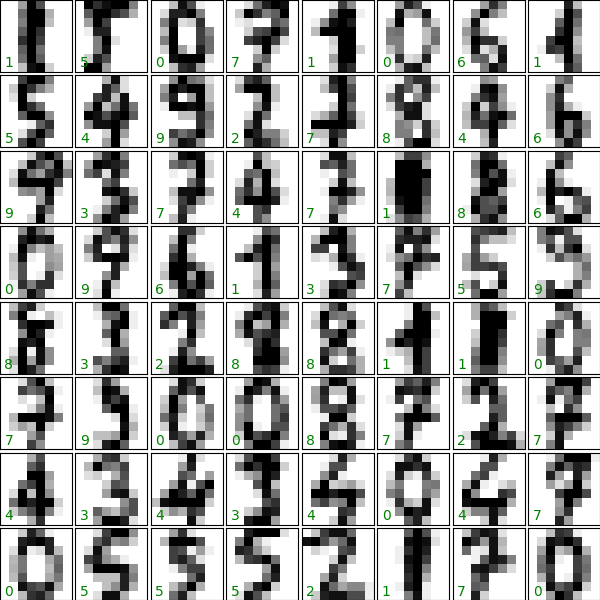# Orthogonal Distance Linear Regression

version 19/3/2018

I implemented a regressor and three classifiers (one binary logistic and two multiclass) based on the Orthogonal Distance algorithm. The methods and attributes are compatible with the scikit-learn Application Programming Interface such that the algorithms here can be used as scikit-learn regressor and classifiers.

The Orthogonal Distance algorithm uses the quasi-chi-squared as the cost function instead of the least-square method. The quasi-chi-squared” converges to conventional chi-square for zero uncertainties in the independent variables x.

The quasi-chi-squared accounts for uncertainties in both independent and dependent variables. The classes are essentially wrappers of the scipy.odr classes, which in turn are wrapper of the Fortran77 rountines in the ODRpack. In contrast to many linear and logistic regression implementation, whose solver is based on the gradient search method, ODRpack uses an anefficient and stable trust region Levenberg-Marquardt procedure.

The routine uses a specifically-designed regularization that scales the coefficients instead of penalizaing the cost function (here the quasi-chi-squared). The parameter C controles the level of regularization with a low value of C corresponding to a high regularization and a high value of C meaning no and small regularization. The package can be download here.

I also provide a couple of examples, mostly derived from scikit-learn. The first example is a logistic fit to the Challenger Space Shuttle O-ring defect data. The incidence of O-ring defects depends on the temperature, which can vary. The Orthogonal Distance Logistic Regression can account for the uncertainty of +/- 2 degree Celsius. The results of the code is shown blow. The  accident occured a day with average temperature of -0.5 centigrads.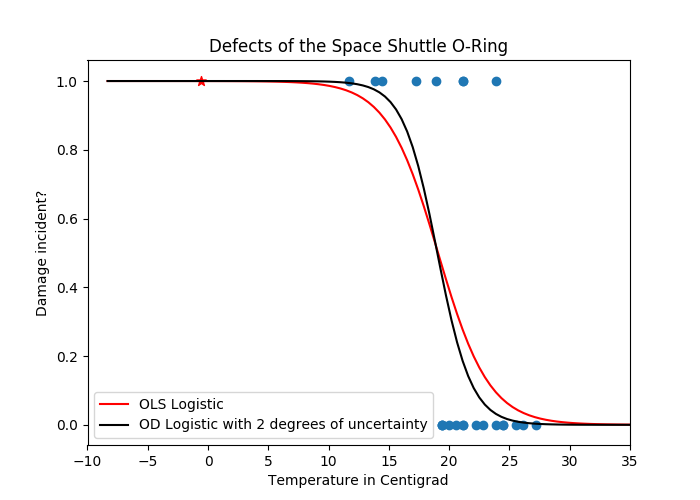The three next examples are toy models for Binary Logistic Classfication (Linearly separable sample, Moon sample, Circle sample).

Linearly separable sample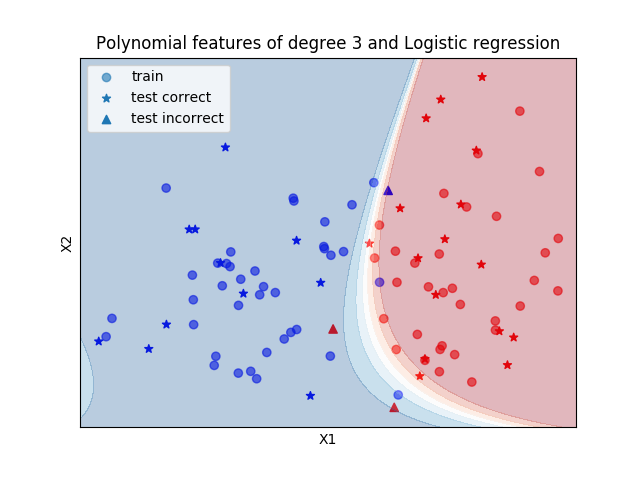Moon sample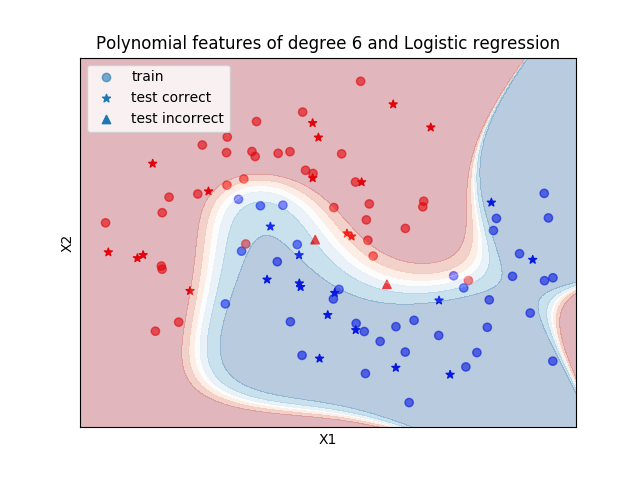Circle sample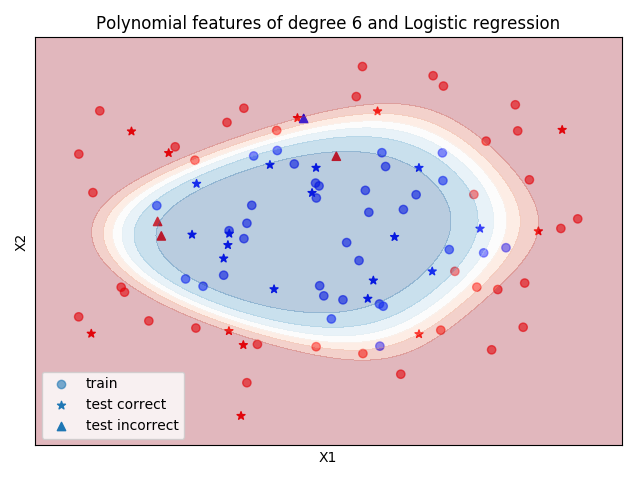The last example shows the multiclass classification method applied to the digit dataset provided in scikit-learn. Here the One-versus-the-Rest algorithm expands the Logisitic binary classification method. The algorithm achieves here an accuracy score of ~0.95.